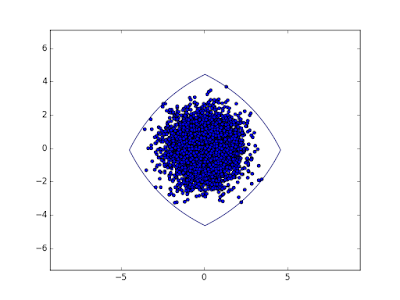## Thursday, May 31, 2018

### Perspective functions (and Luxemburg norms)

If $\varphi:\mathbb{R}_+\to\mathbb{R}$ is a convex function then its perspective function $\tilde{\varphi}:\mathbb{R}_+\times\mathbb{R}_+\to\mathbb{R}$ is defined as
$$\tilde{\varphi}(x,y) = y\varphi(\frac{x}{y}).$$
Moreover, under these conditions, the epigraph of the perspective function
$$\left\{(t,x,y)~:~t\geq y\varphi(\frac{x}{y})\right\}$$
(or, to be precise, its appropriate closure) is a convex cone. Here are some familiar examples:

• $\varphi(x)=x^2$. Then $\tilde{\varphi}(x,y)=\frac{x^2}{y}$, familiar to some as quad-over-lin. The epigraph of $\tilde{\varphi}$, described by $ty\geq x^2$, is the Lorentz cone (rescaled rotated quadratic cone).
• $\varphi(x)=x^p$. Then $\tilde{\varphi}(x,y)=\frac{x^p}{y^{p-1}}$ and the epigraph of $\tilde{\varphi}$, described equivalently by $t^{1/p}y^{1-1/p}\geq |x|$ is the 3-dimensional power cone (with parameter $p$).
• $\varphi(x)=\exp(x)$. Then the epigraph $t\geq y\exp(x/y)$ is the exponential cone.
• $\varphi(x)=x\log(x)$. Then the epigraph $t\geq x\log(x/y)$ is the relative entropy cone.

We bring this up in connection with a series of blogposts by Dirk Lorenz here and here. For a monotone increasing, convex, nonnegative $\varphi$ with  $\varphi(0)=0$ he defines a norm on $\mathbb{R}^n$ via
$$\|x\|_\varphi=\inf\left\{\lambda>0~:~\sum_i\varphi\left(\frac{|x_i|}{\lambda}\right)\leq 1\right\},$$
and we can ask when the norm bound $t\geq \|x\|_\varphi$ is conic representable. The answer is: if the epigraph of the perspective function $\tilde{\varphi}$ is representable, then so is the epigraph of $\|\cdot\|_\varphi$. The reason is that the inequality
$$\sum_i\varphi\left(\frac{|x_i|}{t}\right)\leq 1$$
is equivalent to
$$\begin{array}{ll} w_i\geq |x_i| & i=1,\ldots,n,\\ s_i\geq t\varphi\left(\frac{w_i}{t}\right) & i=1,\ldots,n,\\ t=\sum_i s_i. & \end{array}$$
That covers for example $\varphi(x)=x^2$, $\varphi(x)=x^p$ ($p>1$), $\varphi(x)=\exp(x)-1$, $\varphi(x)=x\log(1+x)$ and so on.Figure: smallest $(\exp(x)-1)$-Luxemburg-norm disk containing a random set of points.

## Wednesday, May 9, 2018

### New Modeling Cookbook

We released a new, revised version of the MOSEK Modeling Cookbook

where you can find practical modeling examples and some theory behind:
• linear optimization,